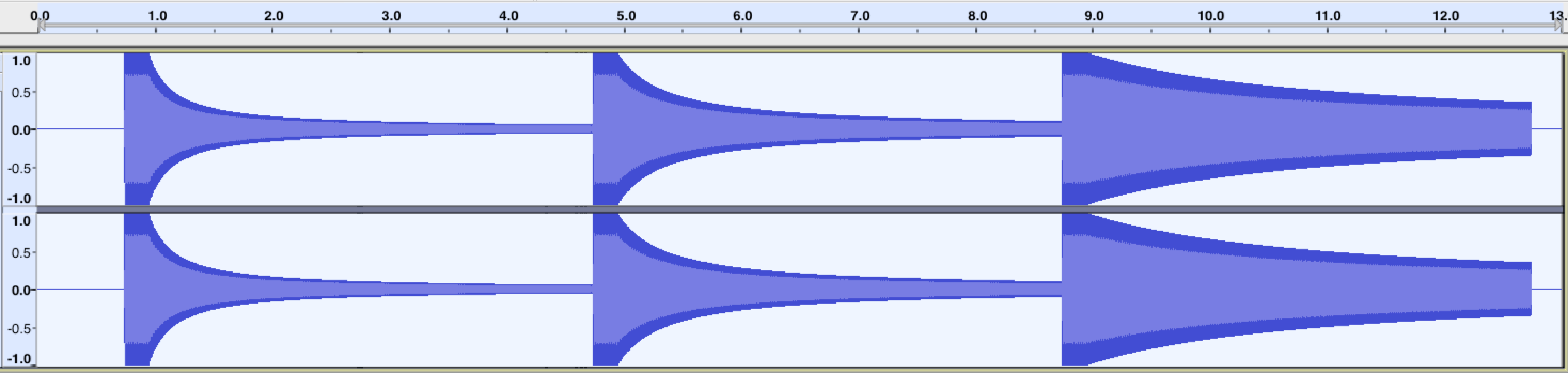# PannerNode.rolloffFactor

The `rolloffFactor` property of the `PannerNode` interface is a double value describing how quickly the volume is reduced as the source moves away from the listener. This value is used by all distance models. The `rolloffFactor` property's default value is `1`.

## Value

A number whose range depends on the `distanceModel` of the panner as follows (negative values are not allowed):

"`linear`"

The range is 0 to 1.

"`inverse`"

The range is 0 to `Infinity`.

"`exponential`"

The range is 0 to `Infinity`.

### Exceptions

`RangeError`

Thrown if the property has been given a value that is outside the accepted range.

## Examples

This example demonstrates how different `rolloffFactor` values affect how the volume of the test tone decreases with increasing distance from the listener:

``````const context = new AudioContext();
// all our test tones will last this many seconds
const NOTE_LENGTH = 4;
// this is how far we'll move the sound
const Z_DISTANCE = 20;

// this function creates a graph for the test tone with a given rolloffFactor
// and schedules it to move away from the listener along the Z (depth-wise) axis
// at the given start time, resulting in a decrease in volume (decay)
const scheduleTestTone = (rolloffFactor, startTime) => {
const osc = new OscillatorNode(context);

const panner = new PannerNode(context);
panner.rolloffFactor = rolloffFactor;

// set the initial Z position, then schedule the ramp
panner.positionZ.setValueAtTime(0, startTime);
panner.positionZ.linearRampToValueAtTime(Z_DISTANCE, startTime + NOTE_LENGTH);

osc.connect(panner)
.connect(context.destination);

osc.start(startTime);
osc.stop(startTime + NOTE_LENGTH);
};

// this tone should decay fairly quickly
scheduleTestTone(1, context.currentTime);
// this tone should decay slower than the previous one
scheduleTestTone(0.5, context.currentTime + NOTE_LENGTH);
// this tone should decay only slightly
scheduleTestTone(0.1, context.currentTime + NOTE_LENGTH * 2);
``````

After running this code, the resulting waveforms should look something like this:## Specifications

Specification
Web Audio API
# dom-pannernode-rollofffactor

## Browser compatibility

BCD tables only load in the browser